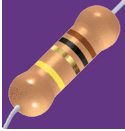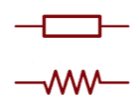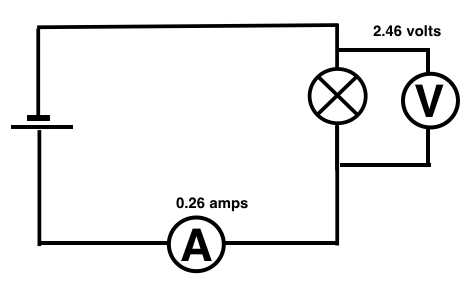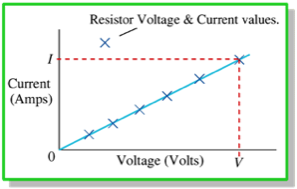ResistanceEvery component in a circuit has a resistance. All tend to obstruct the flow of electricity, although some parts, like a piece of copper wire are very easy for the electricity to flow through. The size of the “obstruction” is called the resistance and it is measured in ohms.  The resistance of a lamp might be around 20 ohms, for a piece of copper wire the resistance is likely to be a tiny fraction of an ohm.

Resistors are put into a circuit just to obstruct the flow of current, perhaps to control the way the current flows around different branches of a circuit. The conventional international symbol for a resistor is the long rectangle, however the zig zag line is still seen in older texts and publications.Measuring resistance

The size of a resistance effects the amount of current flowing through it (since the resistance obstructs the current) and it effects the PD (the voltage) since a larger amount of energy will be required to push the current through a larger resistance.

Resistance cannot be measured directly. We calculate resistance from the ratio of PD to current. The ammeter has to be placed in series with the resistor so that it measures all the current flowing through it. The voltmeter is placed in parallel (around the resistor) therefore measuring the potential difference (PD) from one end to the other.so Resistance = Potential Difference/ Current

in symbols:

R = PD/I   so from the figures above R = 2.46/0.26  = 9.46 ohms

since the measurement is to 2 decimal places the answer should be rounded to 9.5 ohms

P.S. A multimeter seems to measure resistance in one go but in fact the calculation shown above is done by the machine.

Ohm’s Law

Look carefully at the graph below. Note that the ratio of current and voltage is constant because the graph is a straight line. The ratio of PD (volts) / Current (amps) is called the resistance. In a metal this ratio, the resistance is constant, no matter what the values of current and potential difference, providing that the temperature remains constant. This is Ohm’s Law. Although a rather more formal statement goes like this:The current through a metallic conductor is directly proportional to the potential difference (i.e. the voltage) between the two ends, providing that the temperature remains constant.

Ohm’s Law example

Two values taken from the line of best fit is a useful and accurate method of finding an average value of resistance from all of the results.